# Intermediate Geometry : How to find the slope of a perpendicular line

## Example Questions

### Example Question #1 : How To Find The Slope Of A Perpendicular Line

Any line that is perpendicular to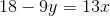must have a slope of what?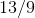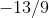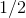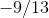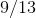Explanation:

Two lines are perpendicular if and only if their slopes are negative reciprocals of each other. To find the slope, we must put the equation into slope-intercept form,, whereequals the slope of the line. First, we must subtractfrom both sides of the equation, giving us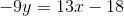. Next, we must divide both sides by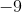, giving us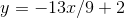. We can now see that the slope of this line is. Therefore, any line that is perpendicular to this one must have a slope of.

### Example Question #2 : How To Find The Slope Of A Perpendicular Line

What is the slope of any line perpendicular to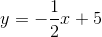?Explanation:

The slope of a perpendicular line is the negative reciprocal of the original slope.

The original slope is.

The negative reciprocal of the original slope is: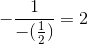### Example Question #3 : How To Find The Slope Of A Perpendicular Line

What is the slope of a line perpendicular to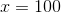?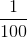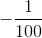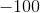Explanation:

The equationis a vertical line on the coordinate plane. Any line perpendicular to a vertical line is a horizontal line. Horizontal lines have slopes of zero.

The correct answer is.

### Example Question #4 : How To Find The Slope Of A Perpendicular Line

Assume the points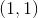and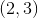form a line. A perpendicular line is drawn to intersect this line. What is the slope of the perpendicular line?Explanation:

Write the slope formula and find the slope of the first line.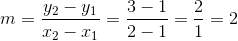The slope of the perpendicular line is the negative reciprocal of the original slope.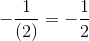Therefore, the slope of the perpendicular line is.

### Example Question #5 : How To Find The Slope Of A Perpendicular Line

A line goes through the following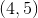and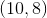.

Find the slope of a line perpendicular to the line given.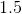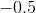Since the given slope is calculated as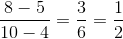, then the slope of the perpendicular line would be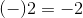.